# Topographic Normalization

## Producer Field Guide

HGD_Product
Producer Field Guide
HGD_Portfolio_Suite
Producer

Digital imagery from mountainous regions often contains a radiometric distortion known as topographic effect. Topographic effect results from the differences in illumination due to the angle of the sun and the angle of the terrain. This causes a variation in the image brightness values. Topographic effect is a combination of:

• incident illumination —the orientation of the surface with respect to the rays of the sun
• exitance angle—the amount of reflected energy as a function of the slope angle
• surface cover characteristics—rugged terrain with high mountains or steep slopes (Hodgson and Shelley, 1994)

One way to reduce topographic effect in digital imagery is by applying transformations based on the Lambertian or Non-Lambertian reflectance models. These models normalize the imagery, which makes it appear as if it were a flat surface.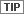Topographic Normalize function uses a Lambertian Reflectance model to normalize topographic effect in Visible to IR imagery.

When using the Topographic Normalization model, enter the following information:

• solar elevation and azimuth at time of image acquisition
• DEM file
• original imagery file (after atmospheric corrections)

Lambertian Reflectance Model

Lambertian Reflectance model assumes that the surface reflects incident solar energy uniformly in all directions, and that variations in reflectance are due to the amount of incident radiation.

The following equation produces normalized brightness values (Colby, 1991; Smith et al, 1980):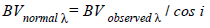Where: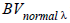= normalized brightness values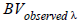= observed brightness values

cos i = cosine of the incidence angle

Incidence Angle

The incidence angle is defined from: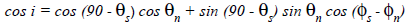Where:

i = angle between the solar rays and the normal to the surface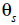= elevation of the sun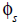= azimuth of the sun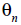= slope of each surface element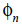= aspect of each surface element

If the surface has a slope of 0 degrees, then aspect is undefined and i is simply 90 -.

Non-Lambertian Model

Minnaert (Minnaert and Szeicz, 1961) proposed that the observed surface does not reflect incident solar energy uniformly in all directions. Instead, he formulated the Non-Lambertian model, which takes into account variations in the terrain. This model, although more computationally demanding than the Lambertian model, may present more accurate results.

In a Non-Lambertian Reflectance model, the following equation is used to normalize the brightness values in the image (Colby, 1991; Smith et al, 1980):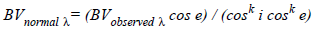Where:= normalized brightness values= observed brightness values

cos i = cosine of the incidence angle

cos e = cosine of the exitance angle, or slope angle

k = empirically derived Minnaert constant

Minnaert Constant

Minnaert constant (k) may be found by regressing a set of observed brightness values from the remotely sensed imagery with known slope and aspect values, provided that all the observations in this set are the same type of land cover. The k value is the slope of the regression line (Hodgson and Shelley, 1994):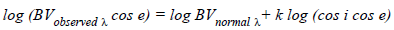Non-Lambertian model does not detect surfaces that are shadowed by intervening topographic features between each pixel and the sun. For these areas, a line-of-sight algorithm can identify such shadowed pixels.

Use Spatial Modeler to create a model based on Non-Lambertian model.